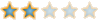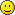# TankDB problem##TankDB problem

i have been trying to fix a problem with the tanks for like 3 months and i cant do it! the problem is the tanks die within 1-5 shots. can someone tell me how to reset the file or tell me what to change

Good SolderPosts : 161
Join date : 2009-02-01
Location : GA##Re: TankDB problem

Code:
`datablock TankData(LightTank : MediumTank){  shapeFile = "~/data/shapes/tanks/tank01.dts";  brainShapeFile = "~/data/shapes/tanks/brainjar01.dts";  maxSpeed = 15.9;          // meters per second (in forward direction)  accelRate = 14.0;        // increase velocity this many meters per second  deccelRate = 20.0;        // when breaking, decrease velocity  turnRate = 61.0;          // degrees per second  antiSlideRate = 10.9;  turretHorRange = 53.5;    // range in +/- degrees of turret horizontally  turretAutoLevel = 0.92;  damageAmount = 11.8;      // total amount of damage tank can withstand  suspensionRange = 0.8;    // proportion of wheel size for wheel to move up/down  damageScale = 1.0;        // multiply damage we do to others by this amount  armorScale = 1.0;        // multiply damage we take by this amount (note: smaller better)  defaultProjectile = "LightProjectile";  ai = GoldLightAI;};`

Make sure the you have these correct:
damageAmount = 11.8;
damageScale = 1.0;

##Re: TankDB problem

tank god ty pinkfloyd!!! i have been changing damage scale only

Good SolderPosts : 161
Join date : 2009-02-01
Location : GA##ok

Code:
`datablock TankData(MediumTank){  category = "Tanks";  shapeFile = "~/data/shapes/tanks/Green/mediumGreenfish.dts";  brainShapeFile = "~/data/shapes/tanks/brainjar01.dts";  camera = "DefaultTankCamera";  fx = "DefaultTankFx";  misc = "DefaultTankMisc";  turnRate = 68.0;          // degrees per second  accelRate = 12.0;        // increase velocity this many meters per second  deccelRate = 20.0;        // when breaking, decrease velocity                            // this many meters per second  coastDeccelRate = 10.0;  // rate of decceleration when coasting...  antiSlideRate = 11.1;      // dissipate sideways velocity by this many meters                            // per second  maxSpeed = 11.9;          // meters per second (in forward direction)  turretVerCenter = 2.0;    // vertical resting angle of turret in degrees  turretHorRange = 58.5;    // range in +/- degrees of turret horizontally  turretVerRange = 21.0;    // range in +/- degrees of turret vertically  turretAutoLevel = 0.5;  suspensionRange = 0.6;    // proportion of wheel size for wheel to move up/down  springRangeX = 0.06;  springRangeY = 0.06;  springVelScale = 1.0;  springCorrectScale1 = 2.0;  springCorrectScale2 = 0.99;  damageAmount = 14.0;      // total amount of damage tank can withstand  damageScale = 1.0;        // multiply damage we do to others by this amount  armorScale = 1.0;        // multiply damage we take by this amount (note: smaller better)  fireScale = 1.0;          // adjust inter-fire interval by scale (2 means twice as slow)  defaultProjectile = "DefaultProjectile";  ai = GoldMediumAI;};datablock TankData(LightTank : MediumTank){  shapeFile = "~/data/shapes/tanks/Green/lightGreenfish.dts";  brainShapeFile = "~/data/shapes/tanks/brainjar01.dts";  maxSpeed = 15.9;          // meters per second (in forward direction)  accelRate = 14.0;        // increase velocity this many meters per second  deccelRate = 20.0;        // when breaking, decrease velocity  turnRate = 61.0;          // degrees per second  antiSlideRate = 10.9;  turretHorRange = 53.5;    // range in +/- degrees of turret horizontally  turretAutoLevel = 0.92;  damageAmount = 11.8;      // total amount of damage tank can withstand  suspensionRange = 0.8;    // proportion of wheel size for wheel to move up/down  damageScale = 1.0;        // multiply damage we do to others by this amount  armorScale = 1.0;        // multiply damage we take by this amount (note: smaller better)  defaultProjectile = "LightProjectile";  ai = GoldLightAI;};datablock TankData(HeavyTank : MediumTank){  shapeFile = "~/data/shapes/tanks/Green/heavyGreenfish.dts";  brainShapeFile = "~/data/shapes/tanks/brainjar01.dts";  accelRate = 8.5;        // increase velocity this many meters per second  deccelRate = 7.85;        // when breaking, decrease velocity  turnRate = 82.0;          // degrees per second  antiSlideRate = 8.25;  //turretHorRange = 53.5;    // range in +/- degrees of turret horizontally  turretHorRange = 68.0;  turretAutoLevel = 1.0;  turretVerRange = 24.0;  maxSpeed = 9.1;          // meters per second (in forward direction)  damageAmount = 21.0;      // total amount of damage tank can withstand  damageScale = 1.0;        // multiply damage we do to others by this amount  armorScale = 1.0;        // multiply damage we take by this amount (note: smaller better)  defaultProjectile = "HeavyProjectile";  ai = GoldHeavyAI;};datablock TankData(BabyBoss : MediumTank){  shapeFile = "~/data/shapes/Boss/puckbot.dts";  brainShapeFile = "~/data/shapes/tanks/brainjar04.dts";  fx = "BabyBossFX";  maxSpeed = 16.0;          // meters per second (in forward direction)  accelRate = 15.0;        // increase velocity this many meters per second  deccelRate = 16.0;        // when breaking, decrease velocity  turnRate = 61.0;          // degrees per second  antiSlideRate = 10.2;  turretHorRange = 33.5;    // range in +/- degrees of turret horizontally  turretAutoLevel = 0.92;  damageAmount = 10.0;      // total amount of damage tank can withstand  suspensionRange = 0.8;    // proportion of wheel size for wheel to move up/down  damageScale = 1.0;        // multiply damage we do to others by this amount  armorScale = 1.8;        // multiply damage we take by this amount (note: smaller better)  defaultProjectile = "LightProjectile";  ai = GoldLightAI;};`

ok man, change the shape file, where it says "shape file"
if you wanna change the medium tank just find

"datablock TankData(MediumTank)"
and then find shapeFile.....

if you wanna change medium to heavy just edit shapeFile to "game/data/shapes/tanks/tank03.dts"
you can change everything you want if you type the path perfect--MTMetal
New TankerPosts : 111
Join date : 2008-12-21
Location : on TGE and youtube, mostly on TT##Re: TankDB problem

i dont get it

Good SolderPosts : 161
Join date : 2009-02-01
Location : GA##Re: TankDB problem

i wanna change TO tanks like light to ufo and boss to med

Good SolderPosts : 161
Join date : 2009-02-01
Location : GA##Re: TankDB problem

Get Codes then.Squishin
BMT MemberPosts : 216
Join date : 2009-01-01##Re: TankDB problem

i have DC and nvm

Good SolderPosts : 161
Join date : 2009-02-01
Location : GA##YOU DON"T GET IT

ok just read the instruction carfully because i have use that and its awesome

##Re: TankDB problem